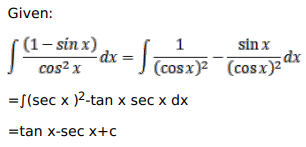# Mark against the correct answer in each of the following:

Question:

Mark $(\sqrt{)}$ against the correct answer in each of the following:

$\int \frac{(1-\sin x)}{\cos ^{2} x} d x=?$

A. $\tan x+\sec x+C$

B. $\tan x-\sec x+C$

C. $-\tan x+\sec x+C$

D. $-\tan x-\sec x+C$

Solution: